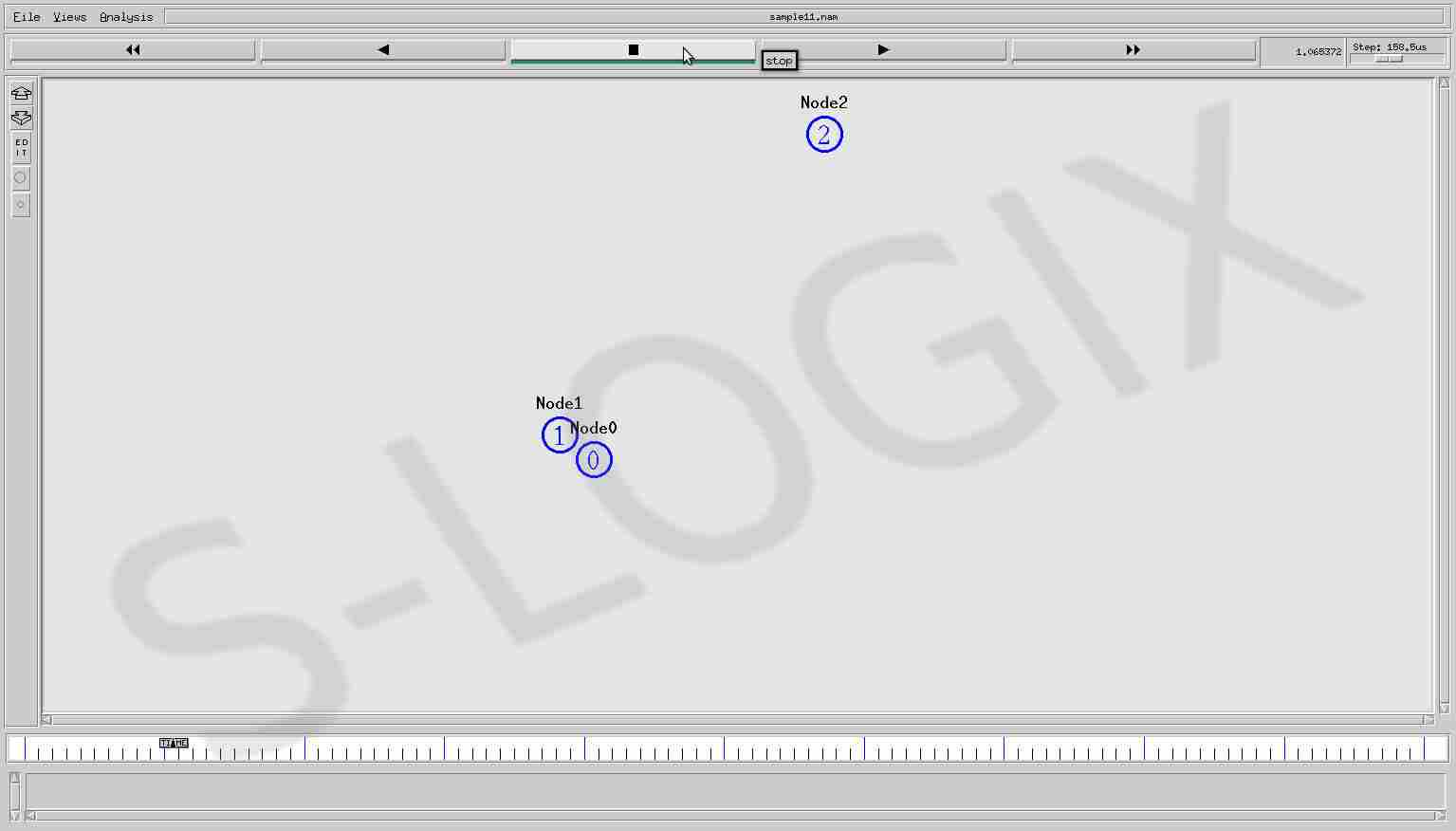• #5, First Floor, 4th Street Dr. Subbarayan Nagar Kodambakkam, Chennai-600 024 Landmark : Samiyar Madam
• pro@slogix.in
• +91- 81240 01111

### How to predict the link failure in ns2?

#### Description

Link breakage prediction in wireless network is necessary to prevent the performance degradation by passing the data over alternative path. Distance is measured at two different instances in the network and the link breakage is predicted from the measured distances. In link_breakage.tcl file, procedure “predict” measures the distance between two nodes and it is called at two different time instances.

###### Sample Code

\$ns at 1.0 "predict"
\$ns at 3.0 "predict"
proc predict {} {
global ns val r node_
set xx(\$i) [\$node_(\$i) set X_]
set yy(\$i) [\$node_(\$i) set Y_]
set xx(\$j) [\$node_(\$j) set X_]
set yy(\$j) [\$node_(\$j) set Y_]
set dx [expr \$xx(\$i) – \$xx(\$j)]
set dy [expr \$yy(\$i) – \$yy(\$j)]
set dx2 [expr \$dx * \$dx]
set dy2 [expr \$dy * \$dy]
set h2 [expr \$dx2 + \$dy2]
set h(\$i-\$j) [expr pow(\$h2, 0.5)]
puts "distance of node(\$i) from node(\$j) = \$h(\$i-\$j)"
puts \$r "distance of node(\$i) from node(\$j) h(\$i-\$j) = \$h(\$i-\$j)"
}

###### Screenshots

Distance measurement at time 1.0

Distance measurement at 3.0